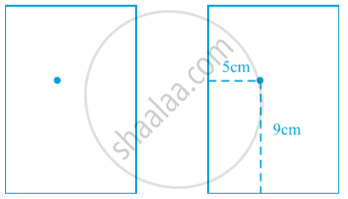# Coordinate Geometry

#### Topics

• To find distance between any two points on an axis.
• To find the distance between two points if the segment joining these points is parallel to any axis in the XY plane.

## Definition

A coordinate geometry is a branch of geometry where the position of the points on the plane is defined with the help of an ordered pair of numbers also known as coordinates. Coordinates are in the form of x and y.

## Notes

The definition of coordinate geometry is the study of algebraic equations on graphs. A coordinate geometry is a branch of geometry where the position of the points on the plane is defined with the help of an ordered pair of numbers also known as coordinates.
For example :
Suppose you put a dot on a sheet of paper in following first fig.The position of the dot on the paper, how will you do this? The dot is in the upper half of the paper”, or “It is near the left edge of the paper”, or “It is very near the left hand upper corner of the sheet”.  The dot is nearly 5 cm away from the left edge of the paper”, it helps to give some idea but still does not fix the position of the dot. . A little thought might enable you to say that the dot is also at a distance of 9 cm above the bottom line.
For this purpose, we fixed the position of the dot by specifying its distances from two fixed lines, the left edge of the paper and the bottom line of the paper in above second Fig. In other words, we need two independent informations for finding the position of the dot.

## Notes

To locate the position of a point on a plane, we require a pair of coordinate axis. The distance of a point from the y-axis is called its x-coordinate, or abscissa. The distance of a point from the x-axis is called its y-coordinate, or x-ordinate. The coordinates of a point on the x-axis are of the form (x, 0), and of a point on the y-axis are of the form (0, y).
You have seen that a linear equation in two variables of the form ax + by + c = 0, (a, b are not simultaneously zero), when represented graphically, gives a straight line.Also,  you have seen the graph of y = ax^2 + bx + c (a ≠ 0), is a parabola.

If you would like to contribute notes or other learning material, please submit them using the button below.

#### Video Tutorials

We have provided more than 1 series of video tutorials for some topics to help you get a better understanding of the topic.

Series 1

Series 2

Series 3

### Shaalaa.com

Coordinate Geometry part 1 (Why coordinate geometry) [00:14:33]
S
0%